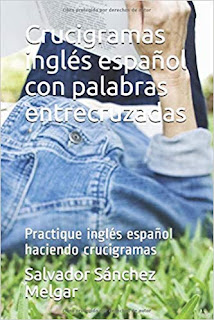Mostrando entradas con la etiqueta 1086 Juego nº 1013 en inglés "Sudoku multiplication Multi 3 x 3". Mostrar todas las entradas
Mostrando entradas con la etiqueta 1086 Juego nº 1013 en inglés "Sudoku multiplication Multi 3 x 3". Mostrar todas las entradas

## sábado, 2 de mayo de 2015

### En inglés "Sudoku multiplication multi 3 x 3" y enlace a mi libro "Sudoku multiplication multi"

Juego nº 1013 al que le he puesto el nombre de "Sudoku multiplication multi 3 x 3" y enlace en inglés de mi libro "Sudoku multiplication multi".

Enlace a mi libro

Introduction Sudoku multiplication
With these sudokus will practice multiplication, develop the mind and memory.
Sudokus multiplication I have named the "MULTI". Game consists of a square with nine squares (3 x 3), which is solved by placing in each of the empty cells without repeating the numbers 1 to 9 so that multiplying together the three numbers in each row must multiply the results exposed in the corresponding right parts; as multiplications of the three numbers in each column, which should be multiplied by the corresponding results shown in the bottom of each column.

"Sudoku multiplication multi 3x3 1 and 2"

Game consists of a square with nine squares (3x3). It resolved placing in each of the empty cells without repeating the numbers 1 to 9 so that multiplying together the three numbers in each row and each column of three numbers must correspond properly with its results exposed to the right of each row and each column below.

Sudoku 1

 =504 1 =18 =40

96            35             108
Solution down

 8 7 9 =504 6 1 3 =18 2 5 4 =40

96          35          108

Sudoku 2

 =144 5 =30 3 =84
144               70                    36
Solution down

 8 2 9 =144 6 5 1 =30 3 7 4 =84
144                 70                     36

Dirección del libro http://www.amazon.com/dp/1511614609
"Sudoku multiplicación" publicado en Amazon el 6/04/2015